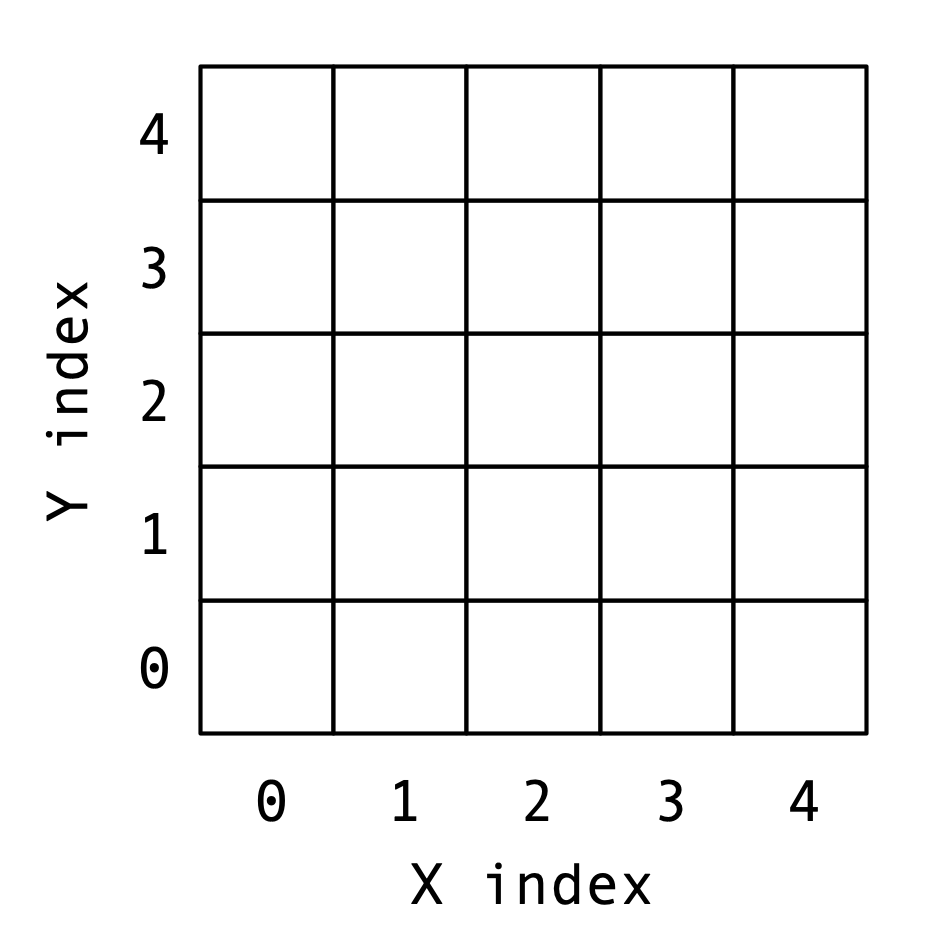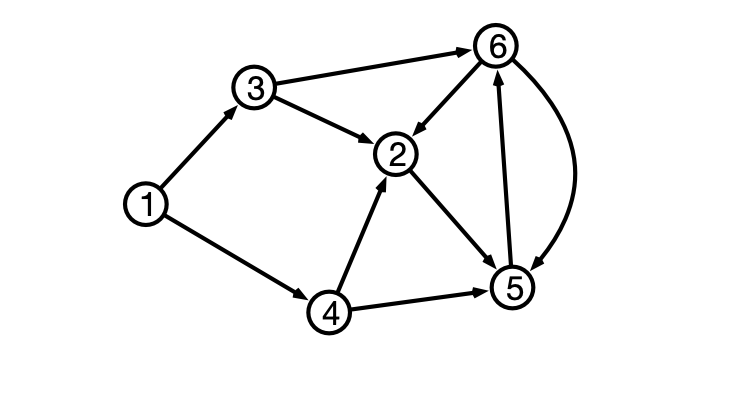# Lecture 26: Sequence Alignment and Shortest Paths

$\def\opt{ {\mathrm{opt}} }$

## Overview

1. Sequence Alignment
2. Shortest Paths, Revisited

## Matching Between Strings

Given strings $X$ and $Y$ form a matching between characters

• matching $M$ is a set of pairs of matched indices

Rules for matching:

• each character is matched with at most one other character
• some characters may be unmatched
• matched characters cannot “cross”
• if $(i, j)$, $(i’, j’)$ are matched with $i < i’$, then $j < j’$

## Sequence Alignment Problem

Input:

• Sequences $X$ and $Y$ of characters of length $n$ and $m$, respectively
• Penalties $\delta, \alpha$ for omission/mismatch

Output:

• A matching $M$ between indices of $X$ and $Y$
• $M$ minimizes total penalty of matching

## An Observation

Suppose

• $X$ sequence of length $n$
• $Y$ sequence of length $m$
• $M$ a matching between $[1, n]$ and $[1, m]$

Claim. Then at least one of the following holds:

1. $(n, m)$ is in $M$
2. $n$ is unmatched in $M$
3. $m$ is unmatched in $M$

Why?

## A Recursive Solution?

Idea. Use previous claim to give recursive characterization of optimal alignment.

How?

Define

• $\opt(i, j) =$ minimum penalty of aligning $X[1..i]$ and $Y[1..j]$
• $M_{i,j}$ is minimum penalty matching between $X[1..i]$ and $Y[1..j]$
• by claim, there are three cases
1. $(i, j) \in M_{i, j}$
2. $i$ unmatched in $M_{i, j}$
3. $j$ unmatched in $M_{i, j}$

## Recursive Solution?

Question. What is a recurrence relation for $\opt(i, j)$?

## Iterative Solution

Construct a two dimensional array p[0..n, 0..m]

• p[i, j] should store $\opt(i, j)$

Question 1. How to initialize p?

Question 2. How to fill out p?## Example

• $X = [R, I, T, E]$
• $Y = [T, I, E, R]$
• $\delta = \alpha = 1$

## Algorithm Pseudocode

  Alignment(X, Y, a, d):
p <- 2d array of dimension (n+1) x (m+1)
for i from 0 to n, p[i, 0] <- i * d
for j from 0 to m, p[0, j] <- j * d
for i from 1 to n
for j from 1 to m
unmatchX <- p[i-1, j] + d
unmatchY <- p[i,j-1] + d
match <- p[i-1,j-1]
if X[i] != Y[j] then match <- match + a
p[i, j] <- Min(unmatchX, unmatchY, match)
return p[n, m]


Running time?

## Conclusion

Optimal alignment between strings can be found in $O(n m)$ time where strings have lengths $n$ and $m$, respectively.

# Shortest Paths, Revisited

## Directed Graphs and Paths## Representing Directed Graphs

• $v$’s neighbors are outgoing neighbors## Previously

Single Source Shortest Paths (SSSP):

Input:

• (Directed) graph $G = (V, E)$, edge weights $w$
• Starting vertex $u$

Output:

• $d(v) =$ distance from $u$ to $v$ for every vertex $v$

## Previous Algorithms

• solves SSSP when all edge weights are $1$
2. Dijkstra’s Algorithm
• solves SSSP when all edge weights are $\geq 0$

Question. What if edge weights can be negative?

## Assumption

Assume. $G$ does not contain any negative weight cycles.

Why?

## Observation

Claim. $G$ a graph with $n$ vertices, $u, v$ vertices in $G$. If $G$ does not contain negative weight cycles, then the shortest (weighted) path from $u$ to $v$ contains at most $n-1$ edges.

Why?

## Intuition

Suppose shortest path from $u$ to $x$ contains $j$ hops.

• $v$ is $x$’s “parent” along path
• $d(u, x) = d(u, v) + w(v, x)$
• shortest path from $u$ to $v$ has $j-1$ hops

## Dynamic Programming Approach

Idea. For each vertex $v$ and each $j = 1, 2, \ldots, n-1$ compute $d_j(u, v) =$ length of shortest path from $u$ to $v$ with at most $j$ hops.

• Note $d(u, v) = d_{n-1}(u, v)$.

## Questions

Question 1. How to initialize $d_0(u, v)$?

Question 2. Given $d_j(u, v)$ for all v, how to find $d_{j+1}(u, v)$?

## Bellman-Ford Algorithm

  Bellman-Ford(V, E, w, u)
d <- 2d array [0..n-1, 1..n]
for v = 1 to n do d[0, v] <- infinity
d[0, u] <- 0
for j = 1 to n-1 do
for each vertex v in V set d[j, v] <- d[j-1,v]
for each vertex v in V
for each neighbor x of v
d[j, x] <- Min(d[j, x], d[j-1, v] + w[v, x])
return d[n-1]


Running time?

Network Flow!